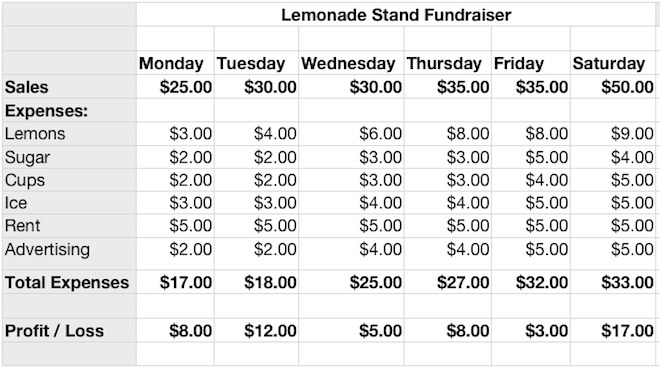iWork Numbers - Lemonade Stand Profit and Loss

Students Create a Profit and Loss Spreadsheet for a Lemonade Stand

Students enter sales and expense data and use formulas to calculate profits or losses for 6 days.

Students learn to save time by using AutoFill to automatically fill in the days of the week, recurring number values (Rent), and repeating formulas (Total Expenses, Profit and Loss).

iWork Numbers Lemonade Stand Profit and Loss Finished Example: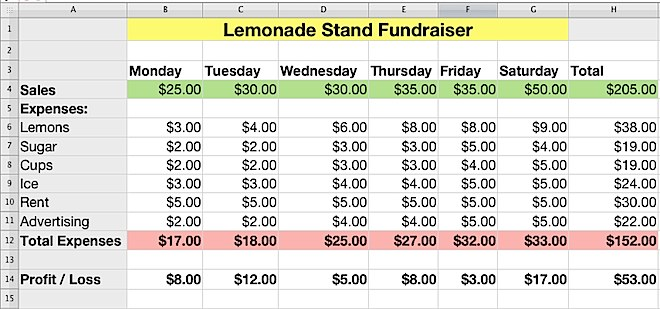Step 1 - Spreadsheet Setup

Enter data as shown. Use autofill to automatically enter weekdays Tues - Sat into the cells. Improve visual appearance by formatting text using larger font size and bold as necessary.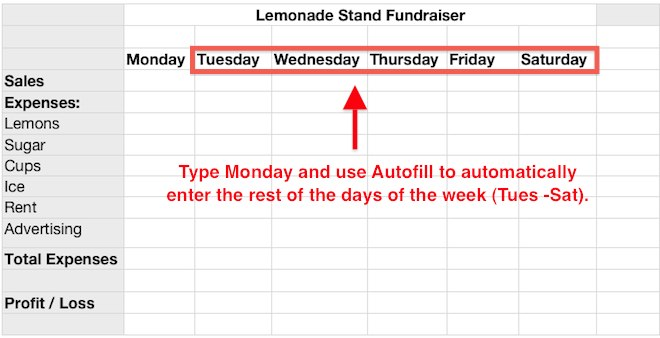20 Second Autofill Tutorial:

Drag the handle (lower right corner) of a selected cell to autofill.

Step 2 - Generate and Enter Sales and Expense Data

Use the Lemonade Stand Sales and Expense Number Generator to quickly generate numbers for each day of the week. Enter the sales and expense data for each day as shown.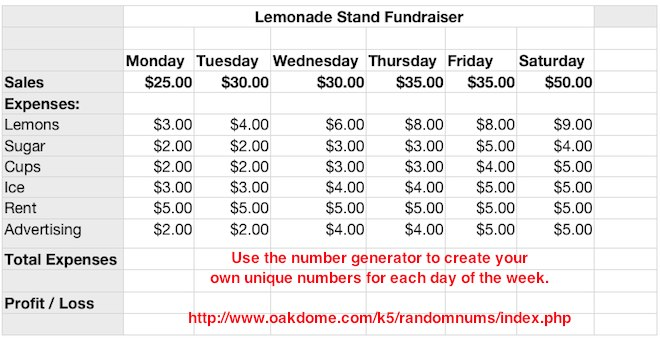Step 3 - Create Formula to Sum Total Expenses

Select all of the expenses in the Monday column as shown. Click the Formula button and click Sum. This will add all the selected expenses and enter the total underneath the selection (\$17.00).

Use Autofill to calculate the total expenses for weekdays Tues - Sat.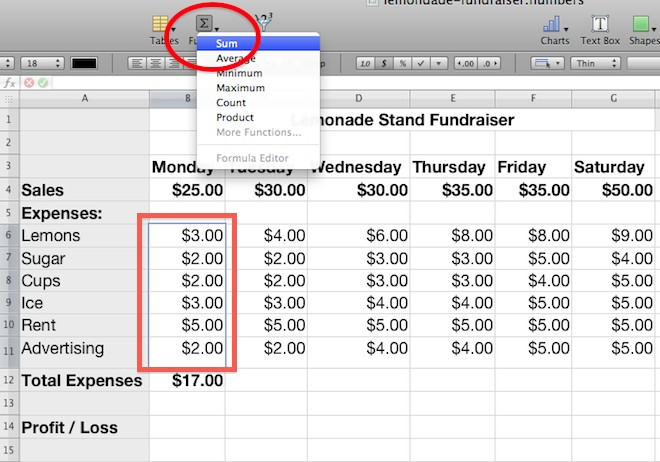Step 4 - Create Formula to Calculate Profit or Loss

Select the cell in the Profit and Loss row in the Monday Column. Enter the equal sign and the cell location of Monday's Sales (b4) minus the cell location of Monday's Total Expenses (b12) as shown below. Click the green checkmark to complete the calculation.

Use Autofill to calculate the profits or losses for weekdays Tues - Sat.Lemonade Stand Finished Example:

Students can format the completed spreadsheet with their name, alternate fonts, font colors, sizes, etc.